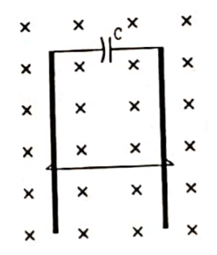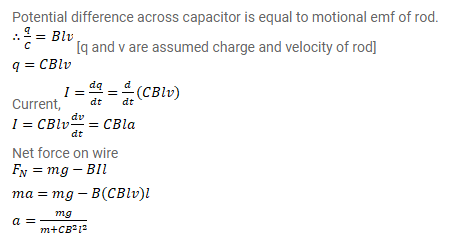# A wire of mass m and length l can slide freely on a pair of smooth,

Question:

A wire of mass $\mathrm{m}$ and length I can slide freely on a pair of smooth, vertical rails. A magnetic field $\mathrm{B}$ exists in the region in the direction perpendicular to the plane of the rails. The rails are connected at the top end by a capacitor of capacitance $C$. Find the acceleration of the wire neglecting any electric resistance.Solution: